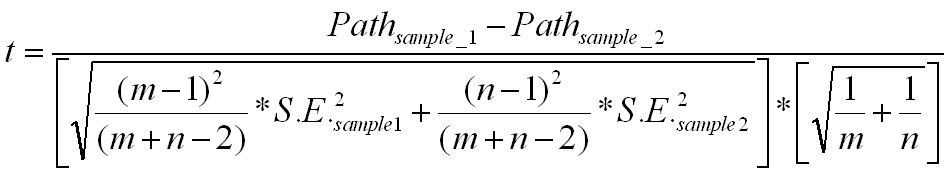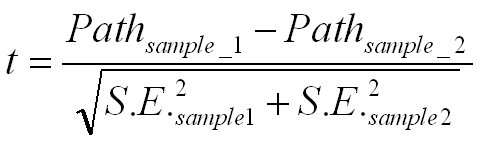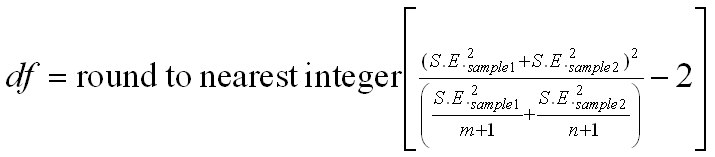Multi-Group analysis with PLS updated 12/21/2004
1. I need some advice. I have a set of husband and wife response to the same questionnaire in a cross-cultural context (i.e. Australian, Chinese, Malay) and I am thinking about the possibilities.
2. Is it possible to treat the husband and wife data as indicators of the same variable in PLSGRAPH? That is instead of combining them manually or something else (e. g. difference scores) I could simply treat it as a part of the outer model. What do you think?

Indeed you could. If both the husband and wife answer the same questions (i.e., the same attitude object), you can treat them as reflective indicators of the same underlying issue. Alternatively, you might even treat them as formative indicators if you view them as capturing different perspectives of an issue. In the case of reflective indicators, there is concern regarding the non-independence of the two measures which may lead to a bias of higher convergence (equivalent to a method effect). Under covariance based procedures, you might model this impact by correlating the error terms for the two indicators. Under PLS (not currently available in PLS-Graph), you could would model a second component.

3.    Could I treat the cross-cultural aspect as a categorical variable in PLSGRAPH or must I do multiple group analysis in LISREL?

You can examine differences in structural across the cultural groupings. It's not automated in PLS-Graph. So, you'd need to take the standard errors for the structural paths provided by PLS-Graph in the re-sampling output and hand calculate it.

The ideal approach would be to do it non-parametrically. I've always planned to develop a permutation approach in PLS-Graph and it is currently under development. This requires you to randomly select cases from the combined multi-group set for each group. Then request the PLS-Graph software to run the samples and print out the results of the re-sampling, sorting the data for each parameter for each population. This is probably way beyond what is expected in current applied papers.

The other approach, which is the most expedient, is to treat the estimates of the re-sampling in a parametric sense via t-tests. You make a parametric assumption and take the standard errors for the structural paths provided by PLS-Graph in the re-sampling output and hand calculate the t-test for the difference in paths between groups.

Essentially, run bootstrap re-samplings for the various groups and treat the standard error estimates from each re-sampling in a parametric sense via t-tests.

You need to calculate the pooled estimator for the variance, which is

Sp =Square root of {[square of (m-1)/(m+n-2)]*square of SE for sample1 + [square of (n-1)/(m+n-2)]*square of SE for sample2}

Then subtract the paths for the two samples. Take this difference and divide by Sp* Square root of (1/m + 1/n)

The complete formula is as follows:This would follow a t-distribution with m+n-2 degrees of freedom.

One caveat in this approach is that there is an assumption that the underlying weights in the formation of constructs for each grouping are approximately equivalent.

Overall, this approach works reasonably well if the two samples are not too non normal and/or the two variances are not too different from one another. If the variance for the two samples are assumed different, a Smith-Satterthwait test can be applied.Unless the N's are large, we need to calculate the degrees of freedom as follows:The only difference between the first and second procedure is the assumption of equal variances for the two populations.  If the variances are equal, the second procedure would be less efficient.  But for large samples, both procedures should yield similar results when the variances are equal.

Note: The formula may differ from standard texts contrasting regression coefficients. The reason for the difference is the use of the bootstrapped standard error. This standard error is already mean adjusted reflecting the standard deviation of the sampling distribution as opposed to the sample or population standard deviation. The formula in many books assume the latter and goes on to adjust by the sample size. So, we need to correct for it by multiplying the SE from the bootstrap by the square root of the sample as well. In other words, (n-1)*square (SE from bootstrap) represents the variance of the sample.